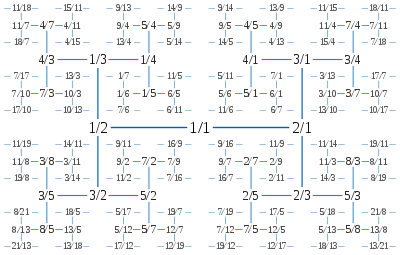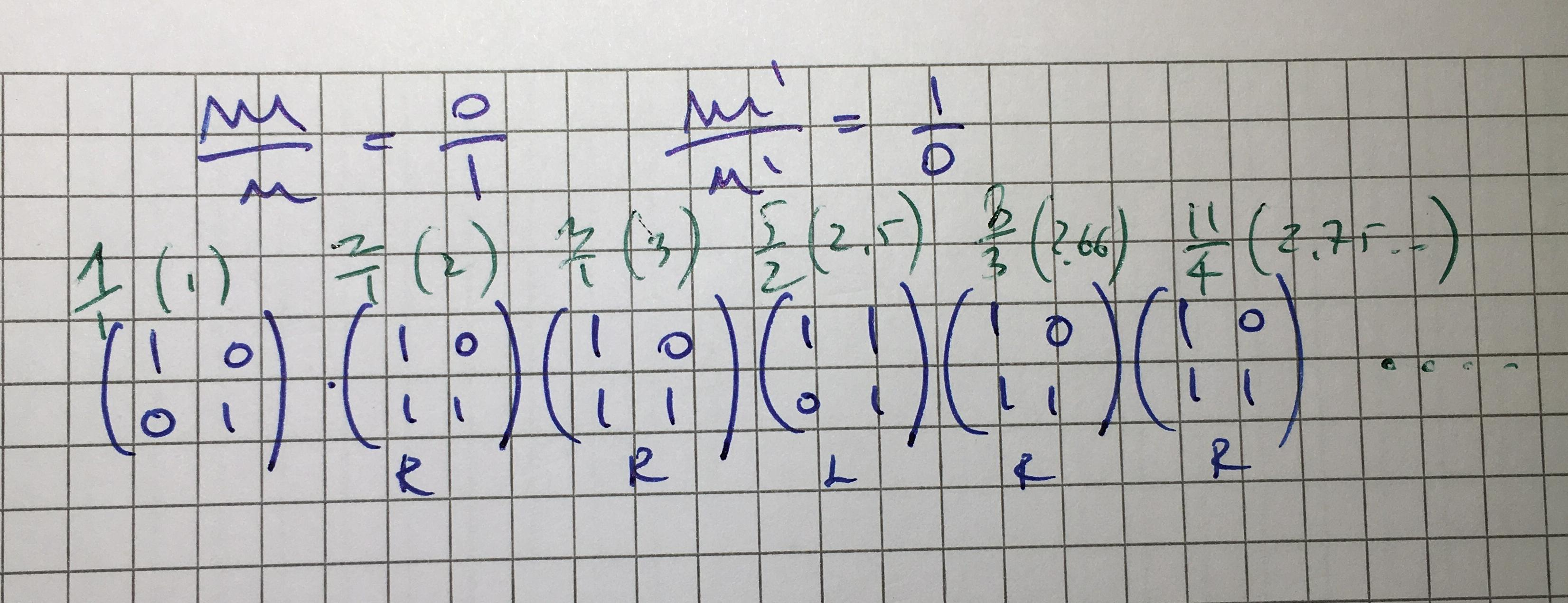# Stern-Brocot tree’s.I’ve encountered this mirable structure this weekend when dealing with cleaver ways to represent fraction of rational numbers n/m where gcd(n,m)=1 (nominator and denominator are relatively prime), Stern-Brocot tree’s are just all about building all the possible fractions that are in they lowest form. What’s interesting (but that came as evident once one understand this structure) is that the SBT (Stern-Brocot tree, from now on) allows to easily build approximation of non rational numbers of any kind, but let’s start with the basic, what we’re talking about?

### Introduction

First, let’s see how to build the SBT, we start with two fractions: 0/1 and 1/0 (Yes I know, but ignore the division by 0), this is from now on what I’m going to call the producing sequence S0, from which we will build S1, S2, … Sn using the following algorithm: Given a sequence of fractions Sn to build Sn+1 let’s put between each consecutive m/n,m’/n’ of the sequence a new rational in the form (m+m’)/(n+n’), that’s it:

S0: 0/1,1/0
S1: 0/1,1/1,1/0
S2: 0/1,1/2,1/1,2/1,1/0
S3: 0/1,1/3,1/2,2/3,1/1,3/2,2/1,3/1,1/0
S4: 0/1,1/4,1/3,2/5,1/2,3/5,2/3,3/4,1/1,4/3,3/2,5/3,2/1,5/2,3/1,4/1,1/0
Sn: …

That is all, but where is the tree? Well, let’s ignore the original generating fractions 0/1 and 1/0 and let’s consider 1/1 as the root of a tree build upon the sequence S4 (This will hold for any Sn),  the size of each sub-sequence at the right and left of the root are equal and are made of an odd number of elements, thus we can again split them in two halves and use the median as new root, recursively repeating this procedure we’ll get the following tree:

That is a very small tree, but you got the idea. You might want to build the tree directly on paper using a more ‘graphical’ approach, in this way the nature of this structure became more clear:Graphical example of how to build the tree. The green arrows are for explanatory purpose, to show from what each node is build on.

Those tree’s have certain very interesting properties, first of all is possible to represent every rational number in the lower terms by using those trees, that means that if you can image a fraction n/m then it will appear somewhere in a SBT big enough. The sequence of numbers appearing as leafs of the three are monotonically increasing, and more generally each Sn is made of increasing fractions where each fraction is unique, there are plenty of other properties for which I suggest to have a look at this book.

You might want to notice one more thing: For each node in the tree it’s right child will have a greater value and it’s left child a smaller value, that is, we’re dealing with a simple binary tree! With such a tree, searching for a rational approximation of an irrational number x is just a matter of binary searching the SBT for the closest n/m to x. But, building all the tree just to find an approximation of is not a great idea, we’re going to have very big SBT’s as we need to reduce the approximation.

Since this is a binary tree and each x as an approximation in it which is reached by a binary search, then we can imagine as a sequence of L or R, where L/R are the direction the binary search algorithm is taking at each step! So what we want is not the entire tree, but only the nodes we need to traverse in order to reach the best approximation available for x, or even better, we would like to build a sequence or L/R that long as we wish, without limitations given by the size of the SBT.

### Looking for the irrational…

Let’s see, we’re looking for x, at any given point it might be smaller or larger than the current root (if it’s equal, then clearly we’re dealing with a rational for which we’ve just found the representation in the SBT), if it’s greater we turn R otherwise L, but what does that mean in mathematical language? Let’s start from 1/1 and let’s see what happen if we attempt to traverse the tree, in order to avoid tons of writing I’ll just put down on paper the case of a single left and right turn, and two right turns from the root:No matter how deep we’re traversing the tree, all the values that are needed at any point are the original m/n and m’/n’.

This help a lot, since all that we need is to ‘select’ the right formula for a turn to right or left on the base of the result of the inequality x<n where n is the current root and x is the irrational we’re looking for. Now is all a problem of correct representation, to quickly calculate the next node value in the tree we need to use some matrices, with very simple manipulations is possible to traverse smoothly the tree and search for more and more accurate representation of irrational, instead of dealing with all that paper writing you’ve seen in the last image.

So let’s start with the original root and see how to represent L and R with matrices:Here we have n/m as left column of the 2×2 matrix, and m’/n’ as right column. Having the fractions represented up side down simplify the calculation.

If you’re unclear about from where the L and R matrices are coming, then just have a look at the previous calculation image from where you can spot immediately the pattern, remember that each column represent the nearest contiguous fractions and the sum of the rows the are the nominator and denominator of the resulting rational. All the problem now is about how we can get from the original matrix to the matrix R or L, well, this is just arithmetic:This is how i get the ‘turning matrices’, which will allow me to traverse the tree without the real need of a tree 🙂

As you can see is all about creating the proper matrix that one can use to transform the ‘origin’ matrix in the left of right matrix. In the image you can see the procedure to find L and R just in case you do not remember how matrix multiplication works.

Now that we know the rules for descending the tree, let’s see how to look for a rational approximation or e:Approximation of e. In green are visible the rationals and their approximate value.

So the process of searching for a number in the three is all about multiplying the original identity matrix with R or L, as I already said, the direction which we’re taking depends on whether the current value of the m/n which is the result of the multiplication is greater or smaller to the searched value, so in the example from the image, since 11/4 is greater than e then the next turn would be left, that means that we need to multiply what we’ve so far with the L matrix. Because e is irrational, then the sequence of turns is infinite and we can get as deep as we want.

### End of the story..

This is all that I wanted to say about the Stern-Brocot tree, there are a lots of other interesting properties and applications that is worth studying about this structure, but this is a blog about programming (mostly) and we’re already going too far with this post. Anyway, you might be interested also in searching for the relation between BST’s and the Farey series, or between SBT’s and the Euclid’s algorithm, but for those better have a look at a good math book.

Hope you’ve enjoyed reading! Thanks!